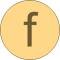# interpolate

Interpolate linearly in a vector# Information

This information is part of the Modelica Standard Library maintained by the Modelica Association.

#### Syntax

```// Real    x[:], y[:], xi, yi;
// Integer iLast, iNew;
yi = Vectors.interpolate(x,y,xi);
(yi, iNew) = Vectors.interpolate(x,y,xi,iLast=1);
```

#### Description

The function call "`Vectors.interpolate(x,y,xi)`" interpolates linearly in vectors (x,y) and returns the value yi that corresponds to xi. Vector x[:] must consist of monotonically increasing values. If xi < x or > x[end], then extrapolation takes places through the first or last two x[:] values, respectively. If the x and y vectors have length 1, then always y is returned. The search for the interval x[iNew] ≤ xi < x[iNew+1] starts at the optional input argument "iLast". The index "iNew" is returned as output argument. The usage of "iLast" and "iNew" is useful to increase the efficiency of the call, if many interpolations take place. If x has two or more identical values then interpolation utilizes the x-value with the largest index.

#### Example

```  Real x1[:] = { 0,  2,  4,  6,  8, 10};
Real x2[:] = { 1,  2,  3,  3,  4,  5};
Real y[:]  = {10, 20, 30, 40, 50, 60};
algorithm
(yi, iNew) := Vectors.interpolate(x1,y,5);  // yi = 35, iNew=3
(yi, iNew) := Vectors.interpolate(x2,y,4);  // yi = 50, iNew=5
(yi, iNew) := Vectors.interpolate(x2,y,3);  // yi = 40, iNew=4
```

# Syntax

(yi, iNew) = interpolate(x, y, xi, iLast)

# Inputs (4)

x Type: Real[:] Description: Abscissa table vector (strict monotonically increasing values required) Type: Real[size(x, 1)] Description: Ordinate table vector Type: Real Description: Desired abscissa value Default Value: 1 Type: Integer Description: Index used in last search

# Outputs (2)

yi Type: Real Description: Ordinate value corresponding to xi Default Value: 1 Type: Integer Description: xi is in the interval x[iNew] <= xi < x[iNew+1]